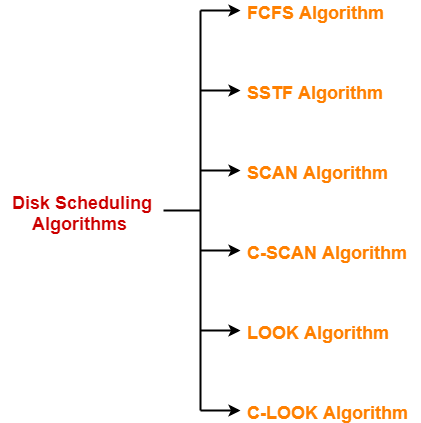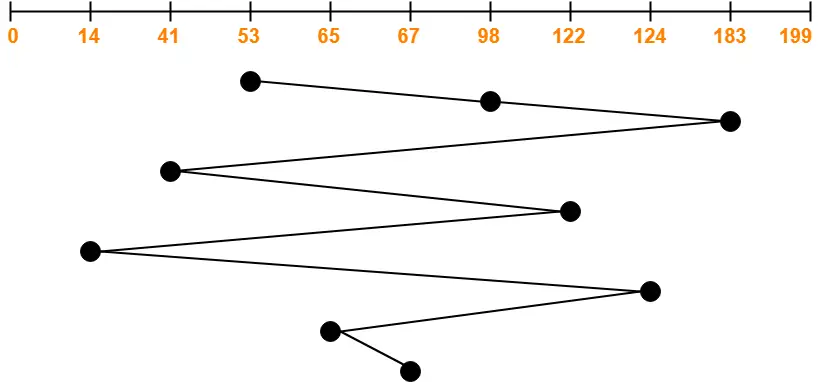## Disk Scheduling-

Before you go through this article, make sure that you have gone through the previous article on Magnetic Disk.

 Disk scheduling is a technique used by the operating system to schedule multiple requests for accessing the disk.

## Disk Scheduling Algorithms-

• The algorithms used for disk scheduling are called as disk scheduling algorithms.
• The purpose of disk scheduling algorithms is to reduce the total seek time.

Various disk scheduling algorithms are-1. FCFS Algorithm
2. SSTF Algorithm
3. SCAN Algorithm
4. C-SCAN Algorithm
5. LOOK Algorithm
6. C-LOOK Algorithm

## FCFS Disk Scheduling Algorithm-

• As the name suggests, this algorithm entertains requests in the order they arrive in the disk queue.
• It is the simplest disk scheduling algorithm.

• It is simple, easy to understand and implement.
• It does not cause starvation to any request.

• It results in increased total seek time.
• It is inefficient.

## Problem-

Consider a disk queue with requests for I/O to blocks on cylinders 98, 183, 41, 122, 14, 124, 65, 67. The FCFS scheduling algorithm is used. The head is initially at cylinder number 53. The cylinders are numbered from 0 to 199. The total head movement (in number of cylinders) incurred while servicing these requests is _______.

## Solution-Total head movements incurred while servicing these requests

= (98 – 53) + (183 – 98) + (183 – 41) + (122 – 41) + (122 – 14) + (124 – 14) + (124 – 65) + (67 – 65)

= 45 + 85 + 142 + 81 + 108 + 110 + 59 + 2

= 632

To gain better understanding about FCFS Disk Scheduling Algorithm,

Watch this Video Lecture

Next Article- SSTF Disk Scheduling Algorithm

Get more notes and other study material of Operating System.

Watch video lectures by visiting our YouTube channel LearnVidFun.